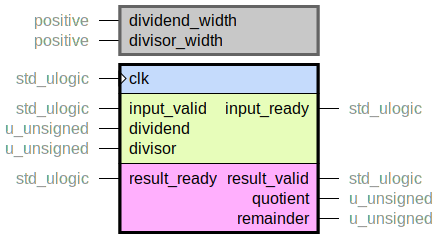# Module math

This document contains technical documentation for the `math` module.

## math_pkg.vhd

Package with some common mathematical functions.

## unsigned_divider.vhdCalculates

dividend / divisor = quotient + remainder / divisor

This is a bit serial divider. Algorithm is the same as long division from elementary school, but with number base two. Latency scales linearly with `dividend_width`.